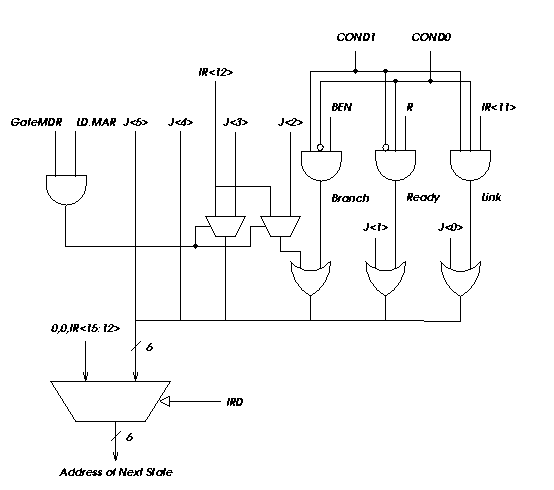Department of Electrical and Computer Engineering
University of Texas

EE 360N
Fall, 1999
Y. N. Patt, Instructor
Problem Set 2
Due: September 27, 1999

1. The Address Control logic allows the same state machine to control memory operations (LD/ST) and I/O operations (in/out). We use LD to do input, and ST to do output. On input, if the address is a device register, the contents of that device register is loaded into MDR using the same state that loads memory into MDR. Similarly, on output, if the address is a device register, the contents of MDR are written to the device register using the same state that stores MDR to memory.

The address control logic combined with the three control signals (MAR, M_EN, and R/W) produce the nine signals that actually control the Memory/I/O subsystem. {Note: as we said in class, M_EN would be better identified as MIO_EN.}

For this exercise, I want you to generate the five input, nine output truth table to control the memory and I/O subsystem. Five inputs because we can decrease the 16-bit MAR to three bits: one bit to distinguish the address as a memory address or a device register address, and two bits to distinguish the four device registers shown in data path. Note for example that if MIO_EN is 0, no Memory/I.O activity is wanted this cycle. What do we do with the nine output signals for such a case? How many of the 32 input combinations are now done! Finish the truth table.

2. We wish a physical memory that has the following attributes: Allowed accesses are 32 bits. Interleaving is eight way. Use 1K by one bit chips. Total memory is 64KB. How many chips are needed? How many address bits are needed? What does each address bit control? Draw a clear picture of this memory, showing clearly the elements discussed in the above questions.

3. The virtual address of variable x is x3456789A. Using the VAX's virtual memory architecture, find the physical address of x.

You will need to know the contents of P0BR: x8AC40000 and SBR: x000C8000.

You will also need to know the contents of physcial memory locations:

x1EBA6EF0: x80000A72
x0022D958:    x800F5D37
a. What virtual page of P0 Space is x on?

b. What is VA of the PTE of the page containing x?

c. What virtual page of System Space is this PTE on?

d. What is the PA of the PTE of this page of System Space?

e. What is the PA of the PTE of the page containing x?

4. It is usually a good idea to combine states from the state machine when it is easy to do. Shown below is a modification to our microsequencer. With it, what states can be combined into a single state, thereby reducing the total number of states. [We are compelled to note that this problem is intended more to test your comprehension of what is going on, than to reflect any real world improvements in the state machine.]5. (Hamacher, pg.255, question 5.20) 1024x1024 array of 32-bit numbers is to be normalized as follows. For each column the largest element is found and all elements of the column are divided by this maximum value.Assume that each page in the virtual memory consists of 4Kbytes and that 1Mbytes of the main memory are allocated for storing data during this computation. Suppose that it takes 40 ms to load a page from the disk to the main memory when a page fault occurs (assume that when we start, the main memory is empty ).

a. How many page faults would occur if the elements of the array are stored in column order in the virtual memory?

b. How many page faults would occur if the elements are stored in row order?

c. Estimate the total time needed to perform this normalization for both arrangements a & b. Assume that it takes 2 ns to do a comparison, 20 ns to do a divide and 100 ns to do a load/store to memory.

6. (Tanenbaum, pg. 201, question 24) A computer with a 32-bit wide data bus uses 1Mx1 dynamic RAM memory chips. What is the smallest memory in bytes that this computer can have?

7. (Patterson & Hennessey, pg. 632, question 7.32) Consider a virtual memory system with the following properties: 40-bit virtual byte address, 16-KB pages, 36-bit physical byte address. What is the total size of the page table for each processor on this machine, assuming that the valid protection dirty and use bits take a total of 4-bits and that all the virtual pages are in use? (Assume that the disc addresses are not stored in the page table).

8. (Patterson & Hennessey, pg. 632, question 7.33) Assume that the virtual memory system of question 7 is implemented with a 2 way set-associative TLB with a total of 256 TLB entries. Show the virtual to physical mapping with a figure like fig. 7.25 on pg. 593. Make sure to label the width of all fields and signals.

9.  We have been referring to the LC-2 memory as 64 Kwords of memory, word-addressible. This is the memory that the user sees, and may bear no relationship to the actual physical memory. Suppose the actual physical address space is 8Kwords, and we keep the notion of 512 word pages. What is  the size of the PFN? Suppose we use the VAX convention of partitioning the physical address space into User Space (P0) and System Space, with 96Kwords of user space and 32 Kwords of system space. Suppose we further insist, like the VAX that System Page Table remains resident in physical memory. If each PTE contained, in addition to the PFN, a Valid bit, a modify bit, and two bits of access control, how many bits of physical memory would be required to store the System Page Table.